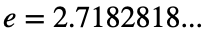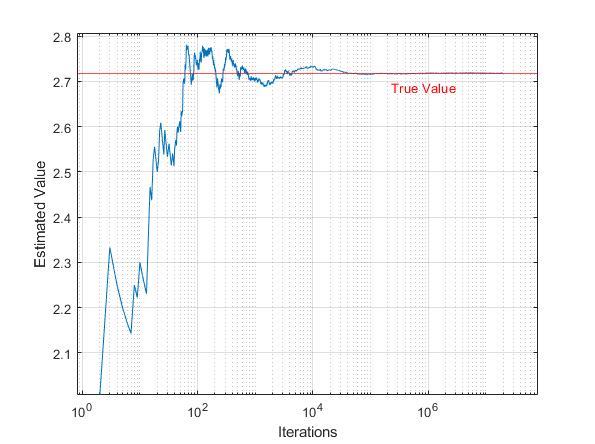# Problem 56000. Estimating Euler's (Oi-ler-z) Number

Euler's number is an irrational constant given byStarting with x=0, count the number of times you can add a uniformly distributed random number to x before it equals or exceeds 1. Repeat this N times, then calculate the estimate of Euler's number by computing the mean of those counts.
The more iterations calcualted, the closer the approximation should be.The input to your function should be the number of iterations of the algorithm to perform (N) and the output should be the estimate of Euler's number.

### Solution Stats

47.27% Correct | 52.73% Incorrect
Last Solution submitted on Nov 22, 2023

### Community Treasure Hunt

Find the treasures in MATLAB Central and discover how the community can help you!

Start Hunting!